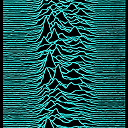Score:5Crypto

# What are the key difference between Shannon entropy and Guessing Entropy?Any body can explain, what are the key differences between Shannon entropy and Guessing Entropy? In some research I got entropy uses binary search or balanced Huffman tree. Guesswork uses linear and unbalances Huffman tree?

Also guesswork can calculate unlimited guessing?Score:3CryptoEdit: Based on the comment of the OP, one way of thinking of the difference between Guesswork and Shannon Entropy is the following:

Shannon: Let's say we have a random variable to encode. Given its distribution, a Huffman code, or other source coding procedure can be used, and the expected length of the codewords in that code is tightly bound to the Shannon entropy of the random variable. A Huffman code tree describes a recursive process where arbitrarily strong queries can be made of the random variable. For example one can ask, for $$X\in {1,\ldots,N\}$$ the question

Is $$X \leq N/3$$?

and pick the left branch of the tree from the root if the answer is yes. In the case of Huffman, we want to minimize the tree and though we use a nice bottom up procedure of "merging" leaves, in the end, if the question is the question above, then $$Pr[X\leq N/3]\approx 1/2.$$ We split left/right at a point that leaves the probabilities of the two subtrees balanced.

Guesswork: In this context, the query cannot be arbitrarily powerful it is typically atomic. What I mean is while building the tree, we are allowed to ask a question of the form

Is $$X=1$$?

for example. Assuming $$Pr[X=1]$$ is the largest atomic probability of the form $$Pr[X=x]$$ where $$x\in \{1,2,\ldots,N\}$$ this is the best question to ask to minimize the number of guesses.In this case you will still have a tree but the cost structure changes, you cannot rule out a subtree if the answer is No you can only rule out a the value $$X=1.$$ This is what leads to Guessing entropy or $$H_{1/2}(X).$$

Applications where this is used include brute force guessing attacks. You cannot ask Is the first letter of a password A? you need to enter the full password and either get in or be rejected. This is why you cannot use atomic questions and ask about parts of the unknown string.

Background:

There has been some discussion here of Guessing entropy (which is really Renyi entropy of order 1/2) in the questions below:

differences between Shannon and Guessing entropies

security of password authentication given entropy

In summary, if you are concerned about the average number of guesses to determine an unknown random variable then you should use Guessing entropy not Shannon entropy since the former is tightly bound to the expected number of guesses.

The Renyi entropy of order $$\alpha$$ is defined by $$H_{\alpha}(X)=\frac{1}{1-\alpha} \log \left(\sum_{x} Pr(X=x)^{\alpha} \right)$$ where $$\alpha \in [0,1) \cup (1,\infty).$$ It obeys $$\lim_{\alpha\rightarrow 1} H_{\alpha}(X)=H(X).$$ It was shown by John Pliam (see here ) that if $$X$$ is a discrete random variable with $$M$$ points in its support $$H(X)$$ can be close to its maximum value $$\log M$$ while the probability of an optimal guessor discovering the actual value of $$X$$ in $$k$$ sequential questions is much less than $$2^{H(X)}=M.$$

Moreover, not only the expected number of guesses, but arbitary moments of the number of guesses can be related to Renyi entropies of various order.

One result on the expectation is that the expected number of guesses to determine a random variable $$X$$ (for the optimal guessing sequence) is upperbounded by

$$2^{H_{1/2}(X)-1}$$

where $$H_{1/2}(X)$$ denotes the Renyi entropy of order $$\alpha=1/2$$ and is also called guessing entropy. Moreover, it is lowerbounded by (for all guessing sequences)

$$\frac{2^{H_{1/2}(X)}}{1 + \log M } \approx 2^{H_{1/2}(X)} / H_{max}(X)$$

where $$H_{max}(X)$$ is the maximum entropy $$\log M$$ in either the Renyi or the Shannon case.Thanks for your answer. But more specifically I wanted to know what is the main scope of use of entropy and guesswork?